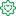Announcements
You can succeed best and quickest by helping others to succeed. Join the conversation.
cancel
Showing results for
Did you mean:Creator

## No sum for expression in pivot chart

I am trying to get this expression to provide the correct sum in a pivot chart.  It shows a sum of zero instead of the correct summed amount.  Any suggestions?

=(If(Match(Account,'Labor','Other ODC','Material/CWT'),

(((Sum(If(Account='Subcontracts/CWTs',([Cost Amount])))
+
Sum(If(Account='Subcontracts/CWTs',[Cost Amount],)
-
Sum(If(Account='Subcontracts/CWTs',([Cost Amount]))))
* [Site Mgmt]

+

(Sum(
If([CCP Description]='Mfg Labor (Mfg Proj Mgr)',[Cost Amount]*[Mfg Labor (Mfg Proj Mgr)],
If([CCP Description]='Mfg Labor (Mfg Engineer)',[Cost Amount]*[Mfg Labor (Mfg Engineer)],
If([CCP Description]='Mfg Labor (Mfg Assistant)',[Cost Amount]*[Mfg Labor (Mfg Assistant)],
If([CCP Description]='Eng Labor (Program Mgr)',[Cost Amount]*[Eng Labor (Program Mgr)],
If([CCP Description]='Eng Labor (Senior Systems Eng)',[Cost Amount]*[Eng Labor (Senior Systems Eng)],
If([CCP Description]='Eng Labor (Systems Eng)',[Cost Amount]*[Eng Labor (Systems Eng)],
If([CCP Description]='Eng Labor (Senior Eng)',[Cost Amount]*[Eng Labor (Senior Eng)],
If([CCP Description]='Eng Labor (Engineer)',[Cost Amount]*[Eng Labor (Engineer)],
If([CCP Description]='Eng Labor (Senior Sftwr Eng)',[Cost Amount]*[Eng Labor (Senior Sftwr Eng)],
If([CCP Description]='Eng Labor (Software Eng)',[Cost Amount]*[Eng Labor (Software Eng)],
If([CCP Description]='Eng Labor (Associate Eng)',[Cost Amount]*[Eng Labor (Associate Eng)],
If([CCP Description]='Eng Labor (Eng Assistant)',[Cost Amount]*[Eng Labor (Eng Assistant)],
If([CCP Description]='Touch Discrete',0,
If(Account='Other ODC',[Cost Amount],
If(Account='Travel ODC',[Cost Amount],
If(Account='Material/CWT',
))))))))))))))))))

+
Sum(
If((Left([CCP Description],9)='Mfg Labor') or Left([CCP Description],14)='Touch Discrete',
(If([CCP Description]='Mfg Labor (Mfg Proj Mgr)',[Cost Amount]*[Mfg Labor (Mfg Proj Mgr)],
If([CCP Description]='Mfg Labor (Mfg Engineer)',[Cost Amount]*[Mfg Labor (Mfg Engineer)],
If([CCP Description]='Mfg Labor (Mfg Assistant)',[Cost Amount]*[Mfg Labor (Mfg Assistant)],
If([CCP Description]='Touch Discrete',[Cost Amount]*[Touch Discrete],Null()
))))
If(Left([CCP Description],9)='Eng Labor',
( If([CCP Description]='Eng Labor (Program Mgr)',[Cost Amount]*[Eng Labor (Program Mgr)],
If([CCP Description]='Eng Labor (Senior Systems Eng)',[Cost Amount]*[Eng Labor (Senior Systems Eng)],
If([CCP Description]='Eng Labor (Systems Eng)',[Cost Amount]*[Eng Labor (Systems Eng)],
If([CCP Description]='Eng Labor (Senior Eng)',[Cost Amount]*[Eng Labor (Senior Eng)],
If([CCP Description]='Eng Labor (Engineer)',[Cost Amount]*[Eng Labor (Engineer)],
If([CCP Description]='Eng Labor (Senior Sftwr Eng)',[Cost Amount]*[Eng Labor (Senior Sftwr Eng)],
If([CCP Description]='Eng Labor (Software Eng)',[Cost Amount]*[Eng Labor (Software Eng)],
If([CCP Description]='Eng Labor (Associate Eng)',[Cost Amount]*[Eng Labor (Associate Eng)],
If([CCP Description]='Eng Labor (Eng Assistant)',[Cost Amount]*[Eng Labor (Eng Assistant)],Null()
))))))))))

If(Account='Material/CWT',0,Null(
))))
))* [Site Mgmt]
),sum(If(Match(Account,'Travel ODC','Subcontracts/CWTs'),0))))

Labels (1)
• ### New To QlikFormer Employee

Have a look at the following Help link for starters:

https://help.qlik.com/en-US/qlikview/April2019/Subsystems/Client/Content/QV_QlikView/ChartFunctions/...

Then you may need to have a look at the following Design Blog post for more info on AGGR:

https://community.qlik.com/t5/Qlik-Design-Blog/Don-t-get-aggr-avated-using-aggr/ba-p/1464136

https://community.qlik.com/t5/Qlik-Design-Blog/Pitfalls-of-the-Aggr-function/ba-p/1463275

Regards,
Brett

To help users find verified answers, please do not forget to use the "Accept as Solution" button on any post(s) that helped you resolve your problem or question.
I now work a compressed schedule, Tuesday, Wednesday and Thursday, so those will be the days I will reply to any follow-up posts.Community Browser